# Area of Composite Figures With Non-Standard Units

Visual number talk prompts that explore the area of a composite figure by decomposing and recomposing with non-standard units.

## In This Set of Math Visual Prompts…

Students will explore area using non-standard square units to determine the area of various composite figures.

## Intentionality…

This set of visual number talk prompts is taken from the Number Talk section of Day 4 in the Make Math Moments Problem Based Unit called Can’t Get Enough Cereal. The purpose of the Number Talk is to reinforce key concepts and big ideas from this problem based math unit including:

• Volume and surface area are attributes of a three-dimensional space
• The volume of a rectangular prism is related to the edge lengths
• The volume of a rectangular prism can be determined by finding the area of the base and multiplying by the number of layers
• Rectangular prisms can be decomposed into measurable parts

Want to take a deeper dive into these concepts? Consider starting from the beginning of this 5-day problem based unit.

## Preparing to Facilitate

Present the visual math talk prompt by playing the video and prompting:

How many squares fit in the figure shown?

Throughout each prompt, students will be asked to determine the value of a figure composed of squares and triangles given the value of a square.

While you could simply leverage an image to display as you prompt students verbally, the following Visual Math Talk Prompts are helpful to Spark Curiosity and lower the floor on these problems so all students can enter into these tasks. Each of the videos reveals the value of the composite figure by decomposing the figure into individual squares and summing their values.

Five (5) additional visual math talk prompts are shared in the Teacher Guide from Day 4 of the Can’t Get Enough Cereal problem based math unit

The following Visual Number Talk Prompt intends to Spark Curiosity and lower the floor so all students can enter into each problem.

Visual Math Talk Prompt #1

Begin playing the video to share the first visual number talk prompt and be ready to pause the video to allow for think time.

Students are encouraged to think about how the shapes can be decomposed, and how the parts relate to one another.

Pause the video where indicated, and share the following prompt:

If the orange square is worth 1 whole, how much is each shape worth?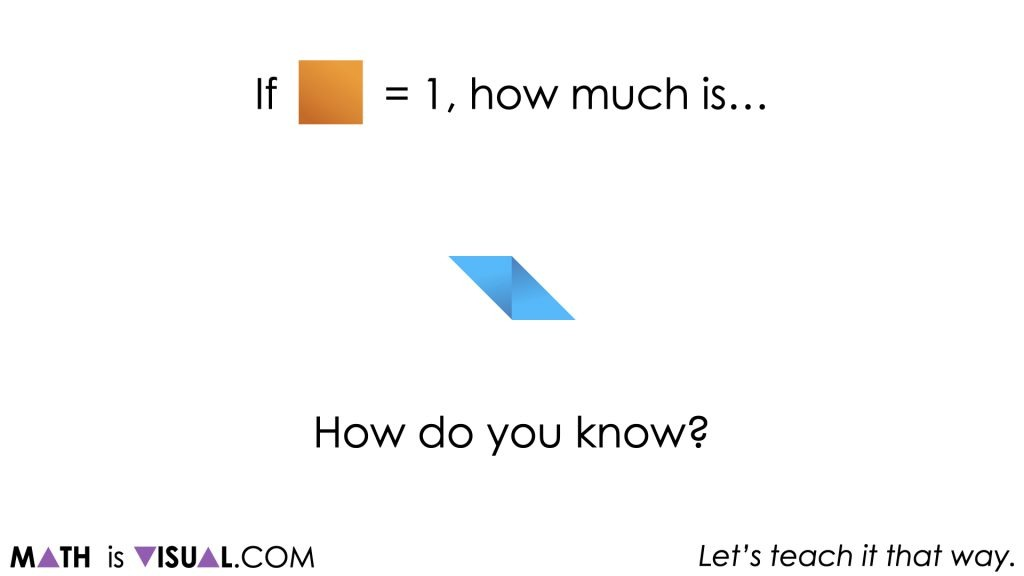Some students may be able to leverage their spatial reasoning skills to quickly conclude that the value of the blue parallelogram is 1, since it can be decomposed and recomposed into a square with the same dimensions as the orange square.

Once you resume playing the video, you’ll see the parallelogram decompose into two right triangles and recompose into a square.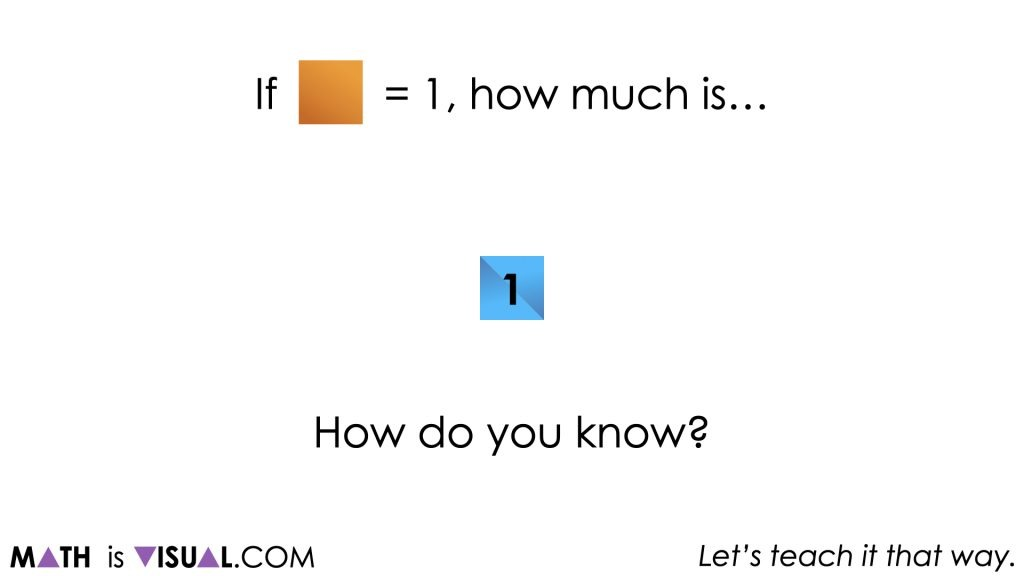Visual Math Talk Prompt #2

Continue playing the video for the next Visual Math Talk Prompt.

In this case, students may quickly notice that this figure is composed of a square wedged between the two right triangles that they encountered in the previous prompt.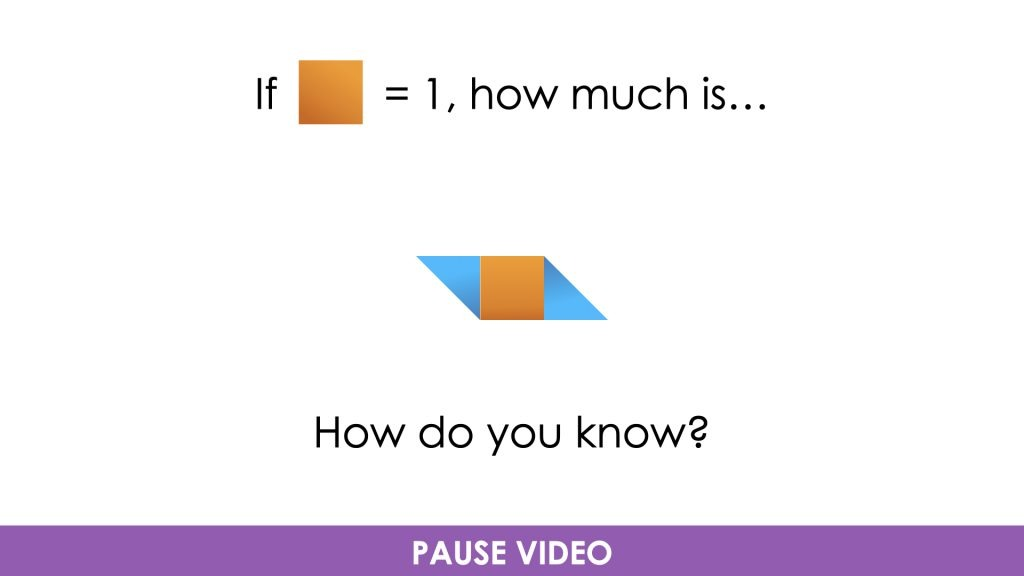Therefore, we can conclude (and the visual math talk prompt video will confirm via animation) that the value of the figure is 2.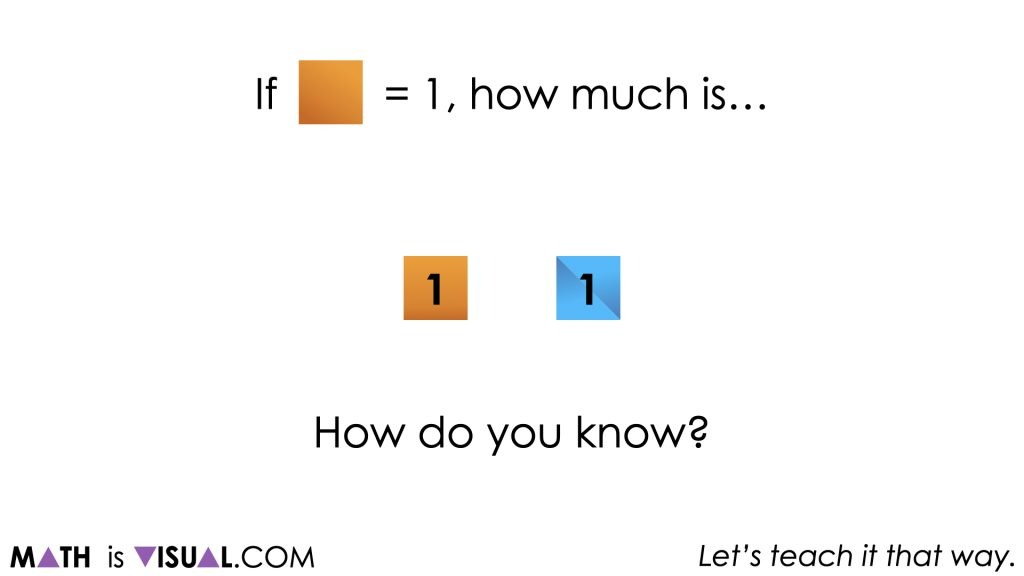Visual Math Talk Prompt #3

Continue playing the Visual Math Talk Prompt video and pause for students to think about the area of the next composite figure: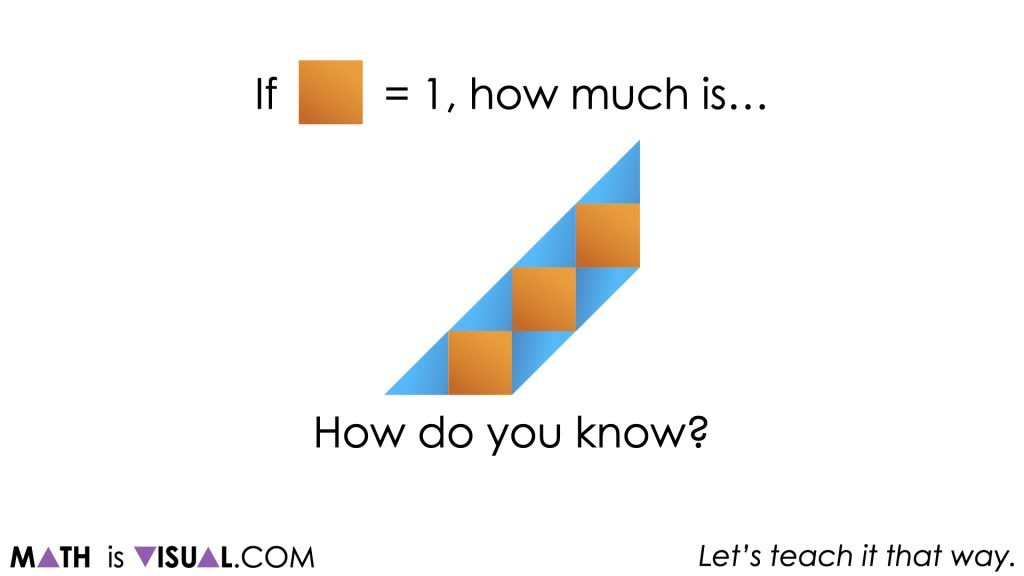Hopefully students will be able to quickly conclude that there are 3 orange squares in this figure and that the 6 right triangles can compose an additional 3 squares for a total value of 6.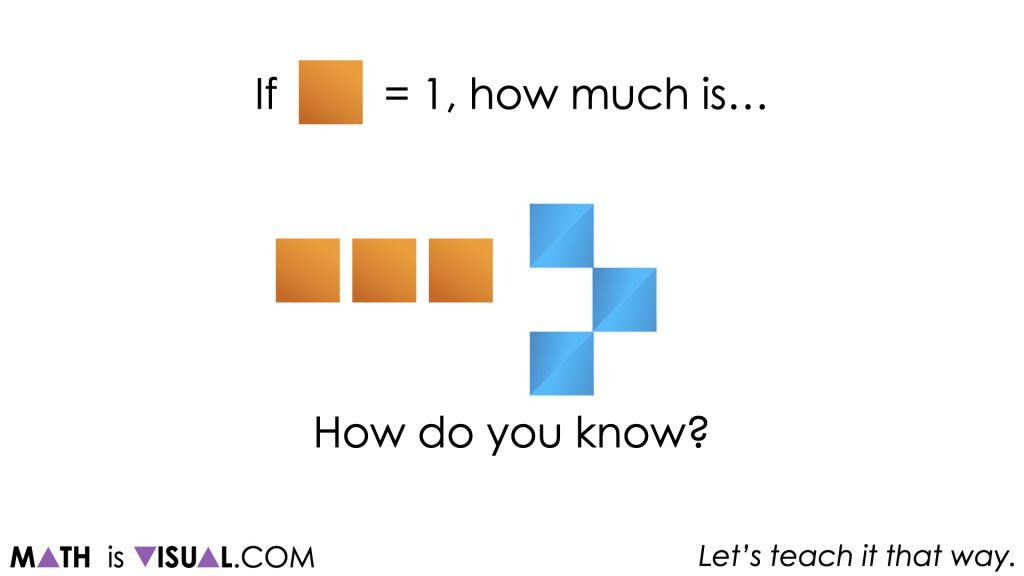## Want to Explore These Concepts & Skills Further?

Five (5) additional number talk prompts are available in Day 4 of the Can’t Get Enough Cereal problem based math unit that you can dive into now.

Why not start from the beginning of this contextual 5-day unit of real world lessons from the Make Math Moments Problem Based Units page.

Did you use this in your classroom or at home? How’d it go? Post in the comments!

Math IS Visual. Let’s teach it that way.